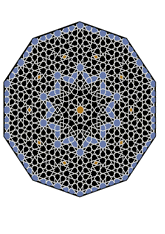data189/MOR0312

## Geometry

• The symmetry group of the tiling is *10.0• (d10.0).
• All the internal angles of the constituent polygons are a multiple of 36°.
• Contains two regular two-pointed star polygons with vertex angle of 72°.
• Contains 8 regular five-pointed star polygons with vertex angle of 36°.
• Contains four regular 10-pointed star polygons with vertex angle of 72°.
• There are 9 non-regular reflective tiles and one reflective pair.
• The tiling is edge-to-edge.
• As drawn, contains about 1051 polygons.

## References

Publications referenced: